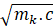# Cosmology, Lie algebras and Koide's formula.

Title: The cosmology as origin of the Koide’s mass formula in particles physics.

French matriculation: ISBN 978-2-36923-008-3, EAN 9782369230083.

Version: 3.

Language: GB/USA.

Number of pages: 9.

Publication: 15 December 2022.

The context.

The Wikipedia article on that item starts with that sentence (citation, 14 December 2019): “The Koide formula is an unexplained empirical equation discovered by Yoshio Koide. In its original form, it relates the masses of the three charged leptons; later authors have extended the relation to neutrinos, quarks, and other families of particles”.

For many physicists, this formula is only understood as “numerology” without serious foundation.

Therefore, some of them have proposed (new citation): “… mechanisms to explain origins of the charged lepton spectrum as well as the Koide formula, e.g., by constructing an effective field theory in which a new gauge symmetry causes the pole masses to exactly satisfy the relation”.

My contribution.

The Theory of the (E) Question (alias: the TEQ) is a set of mathematical explorations focusing its attention on the deformations of tensor products.

This mathematical tool allows the construction of a new scenario explaining the Koide’s formula.

In that context, the Koide’s formula appears to be a natural but peculiar consequence of a quite brighter model.

The main idea relies on the fact that the speed of light must be preserved in any type of vacuum.

Due to the Morley and Michelson experiments, we already knew that it was true for a perfect Maxwell’s vacuum.

My exploration envisages what happens when that speed is preserved in an evolutive FLRW metric if the tensor products are deformed.

The condition describing the preservation contains a family of situations not depending on the spatial metric. In extenso, they only depend on the cubes defining the deformations of tensor products.

Any cube which is involved in that exploration is the superposition of three (3-3) matrices in M(3, C). I look for matrices respecting the condition preserving the speed of light in any empty region of the universe.

After some tries and cumbersome algebraic manipulations (only visible in the French version of this document), I discover (what I call Perian) representations doing the job.

[A] = λ.Id3 + μ.T2(⊗)(q, q) + θ.[J]Φ(q)

I also state that, under certain conditions, the exponential map of these representations can be Euler-Rodrigues parametrizations related to quaternions (q0a, qa):

[Q] = exp([A])

Furthermore, when:

• a deforming cube A (respecting the condition preserving the condition preserving the speed of light) is the superposition of three Perian representations and
• the Euler-Rodrigues parametrization associated with each of these matrices represents a unit quaternion with a vanishing real component (0, qa) in such a manner that the three vectors (q1, q2, q3) form a normed orthogonal basis,

… then the Koide’s ratio of each vector qa is 2/3.

Hence, if the components of these vectors can be related to the mass of particles, for example through the relation:

qak =Then, the scenario which I propose in my document introduces the Koide’s mass formula.

© Thierry PERIAT, 15 December 2022.

Go back to the page:

Date de dernière mise à jour : 19/12/2022

• Aucune note. Soyez le premier à attribuer une note !